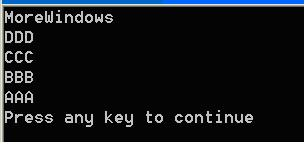## C++ 类的静态成员详细讲解

为什么80%的码农都做不了架构师？->>>在C++中，静态成员是属于整个类的而不是某个对象，静态成员变量只存储一份供所有对象共用。所以在所有对象中都可以共享它。使用静态成员变量实现多个对象之间的数据共享不会破坏隐藏的原则，保证了安全性还可以节省内存。

```class Point
{
public:
void init()
{
}
static void output()
{
}
};
void main()
{
Point::init();
Point::output();
}```

```void main()
{
Point pt;
pt.init();
pt.output();
}```

```#include <stdio.h>
class Point
{
public:
void init()
{
}
static void output()
{
printf("%d\n", m_x);
}
private:
int m_x;
};
void main()
{
Point pt;
pt.output();
}```

```class Point
{
public:
void init()
{
output();
}
static void output()
{
}
};
void main()
{
Point pt;
pt.output();
}```

```#include <stdio.h>
class Point
{
public:
Point()
{
m_nPointCount++;
}
~Point()
{
m_nPointCount--;
}
static void output()
{
printf("%d\n", m_nPointCount);
}
private:
static int m_nPointCount;
};
void main()
{
Point pt;
pt.output();
}```

error LNK2001: unresolved external symbol "private: static int Point::m_nPointCount" (?m_nPointCount@Point@@0HA)

```#include <stdio.h>
#include <string.h>
const int MAX_NAME_SIZE = 30;

class Student
{
public:
Student(char *pszName);
~Student();
public:
static void PrintfAllStudents();
private:
char    m_name[MAX_NAME_SIZE];
Student *next;
Student *prev;
};

Student::Student(char *pszName)
{
strcpy(this->m_name, pszName);

//建立双向链表，新数据从链表头部插入。
this->prev = NULL;
if (m_head != NULL)
}

Student::~Student ()//析构过程就是节点的脱离过程
{
if (this == m_head) //该节点就是头节点。
{
}
else
{
this->prev->next = this->next;
this->next->prev = this->prev;
}
}

void Student::PrintfAllStudents()
{
for (Student *p = m_head; p != NULL; p = p->next)
printf("%s\n", p->m_name);
}

Student* Student::m_head = NULL;

void main()
{
Student studentA("AAA");
Student studentB("BBB");
Student studentC("CCC");
Student studentD("DDD");
Student student("MoreWindows");
Student::PrintfAllStudents();
}```0
h